# NCERT Solutions for Class 6 Maths Chapter 7 Fractions

## NCERT Solutions for Class 6 Maths Chapter 7 Fractions

Today in our article you will be told that when your ncert solutions for class 6 maths Chapter 7 Fractions will be released and you will be provided complete information about it. In our article, you will also be given complete information about the model paper. Also, you will be provided complete information about the model paper download process in our article. So please read it carefully till the end and stay connected with our website till the end.

ncert solutions for class 6 maths Chapter 7 Fractions

## What are the crucial topics covered in the NCERT Solutions for Class 6 Maths Chapter 7?

The crucial topics covered in the NCERT Solutions for Class 6 Maths Chapter 7 are –
7.1 – Introduction
7.2 – A Fraction
7.3 – Fraction on the Number Line
7.4 – Proper Fractions
7.5 – Improper and Mixed Fractions
7.6 – Equivalent Fractions
7.7 – Simplest Form of a Fraction
7.8 – Like Fractions
7.9 – Comparing Fractions
7.10 – Addition and Subtraction of Fractions

## Exercise 7.1

1. Write the fraction representing the shaded portion.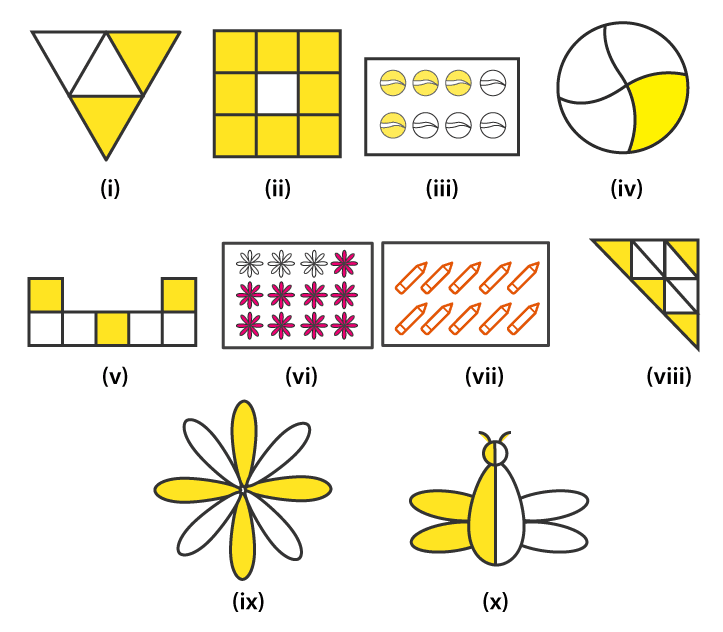Solutions:

(i) Number of parts = 4

∴ Fraction = 2 / 4

(ii) Number of parts = 9

∴ Fraction = 8 / 9

(iii) Number of parts = 8

∴ Fraction = 4 / 8

(iv) Number of parts = 4

∴ Fraction = 1 / 4

(v) Number of parts = 7

∴ Fraction = 3 / 7

(vi) Number of parts = 12

∴ Fraction = 3 / 12

(vii) Number of parts = 10

∴ Fraction = 10 / 10

(viii) Number of parts = 9

∴ Fraction = 4 / 9

(ix) Number of parts = 8

∴ Fraction = 4 / 8

(x) Number of parts = 2

∴ Fraction = 1 / 2

2. Colour the part according to the given fraction.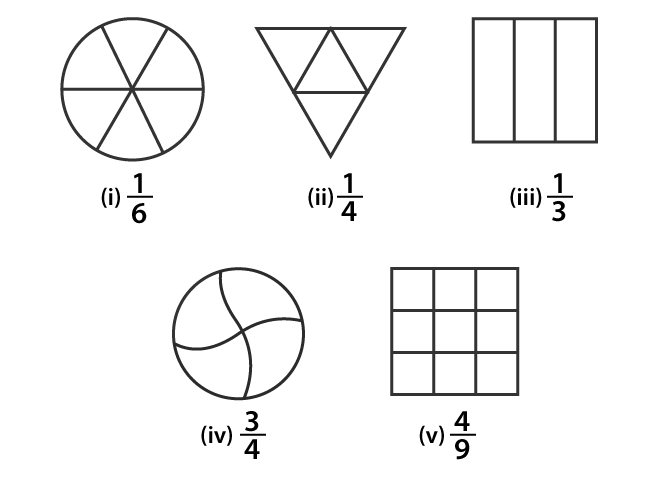Solutions: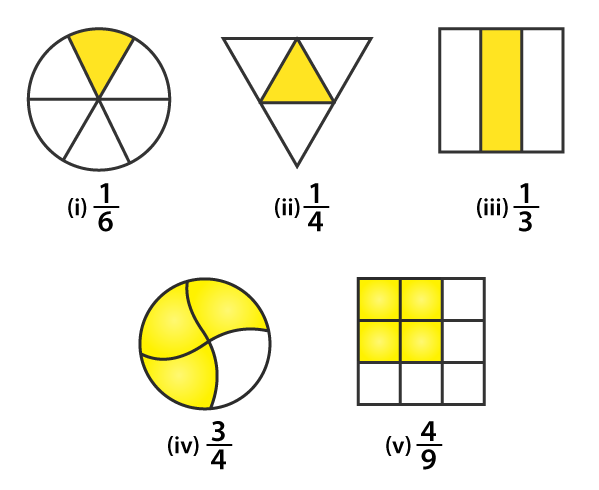3. Identify the error, if any.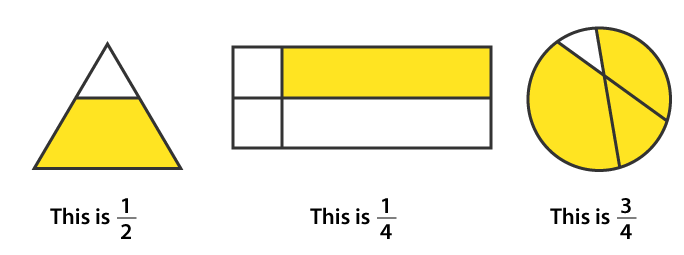Solutions:

(i) The shaded portion is not half

Hence, this is not 1 / 2

(ii) Since, the parts are not equal

∴ The shaded portion is not 1 / 4

(iii) Since, the parts are not equal

∴ The shaded portion is not 3 / 4

4. What fraction of a day is 8 hours?

Solutions:

There are 24 hours in a day

We have 8 hours

Hence, the required fraction is 8 / 24

5. What fraction of an hour is 40 minutes?

Solutions:

There are 60 minutes in 1 hour

∴ 1 hour = 60 minutes

Hence, required Fraction = 40 / 60

6. Arya, Abhimanyu, and Vivek shared lunch. Arya has brought two sandwiches, one made of vegetables and one of jam. The other two boys forgot to bring their lunch. Arya agreed to share his sandwiches so that each person will have an equal share of each sandwich.

(a) How can Arya divide his sandwiches so that each person has an equal share?

(b) What part of a sandwich will each boy receive?

Solutions:

(a) Arya has divided the sandwich into 3 equal parts. So each person will get one part.

(b) Each boy receive 1 / 3 part

∴ Required Fraction is 1 / 3

7. Kanchan dyes dresses. She had to dye 30 dresses. She has so far finished 20 dresses. What fraction of dresses has she finished?

Solutions:

Total number of dresses Kanchan has to dye = 30 dresses

Number of dresses she has finished = 20 dresses

∴ Required Fraction = 20 / 30 = 2 / 3

8. Write the natural numbers from 2 to 12. What fraction of them are prime numbers?

Solutions:

Natural numbers from 2 to 12 are

2, 3, 4, 5, 6, 7, 8, 9, 10, 11, 12

Total number of natural numbers given= 11

Number of prime numbers = 5

∴ Required Fraction = 5 / 11

9. Write the natural numbers from 102 to 113. What fraction of them are prime numbers?

Solutions:

Natural numbers from 102 to 113 are

102, 103, 104, 105, 106, 107, 108, 109, 110, 111, 112, 113

Total number of natural numbers given = 12

Number of prime numbers = 4 [103, 107, 109, 113]

∴ Required Fraction = 4 / 12 = 1 / 3

10. What fraction of these circles has X’s in them?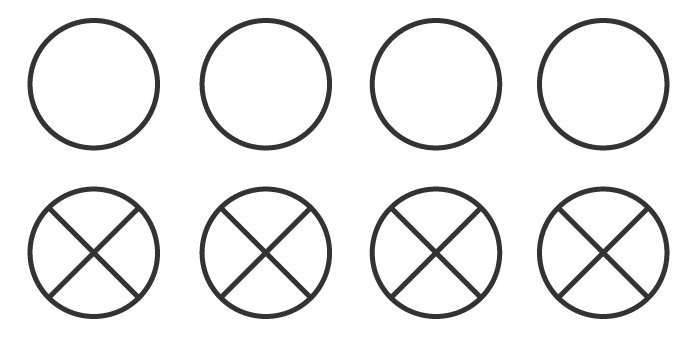Solutions:

Total number of circles in the figure = 8

Number of circles having X’s in them = 4

∴ Required Fraction = 4 / 8 = 1 / 2

11. Kristin received a CD player for her birthday. She bought 3 CDs and received 5 others as gifts. What fraction of her total CDs did she buy and what fraction did she receive as gifts?

Solutions:

Number of CDs Kristin bought from the market = 3

Total number of CDs Kristin have = 3 + 5 = 8

∴ Fraction of CD she bought = 3 / 8

## Exercise 7.2

1. Draw number lines and locate the points on them:

(a) 1 / 2, 1 / 4, 3 / 4, 4 / 4

(b) 1 / 8, 2 / 8, 3 / 8, 7 / 8

(c) 2 / 5, 3 / 5, 8 / 5, 4 / 5

Solutions:

(a) 1 / 2, 1 / 4, 3 / 4, 4 / 4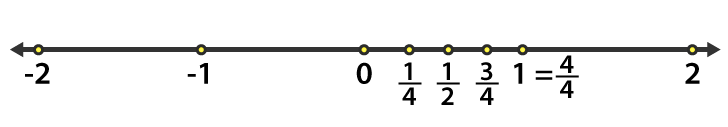Here divide the number line from 0 to 1 into four equal parts

C = 2 / 4 = 1 / 2

B = 1 / 4

D = 3 / 4 and

E = 4 / 4 = 1

(b) 1 / 8, 2 / 8, 3 / 8, 7 / 8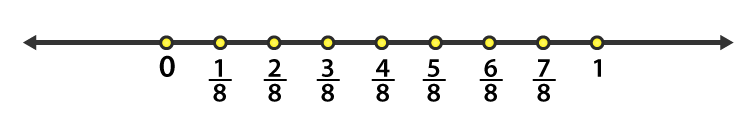Divide the number line from 0 to 1 into eight equal parts

B = 1 / 8

C = 2 / 8

D = 3 / 8

H = 7 / 8

(c) 2 / 5, 3 / 5, 8 / 5, 4 / 5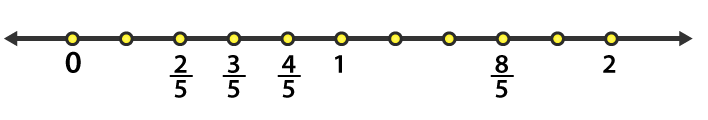From the given number line, we have

C = 2 / 5

D = 3 / 5

E = 4 / 5

I = 8 / 5

2. Express the following as mixed fractions:

(a) 20 / 3

(b) 11 / 5

(c) 17 / 7

(d) 28 / 5

(e) 19 / 6

(f) 35 / 9

Solutions:

(a) 20 / 3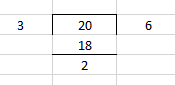∴ 20 / 3 =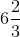(b) 11 / 5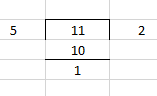∴ 11 / 5 =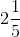(c) 17 / 7

∴ 17 / 7 =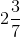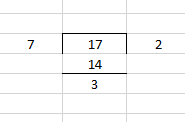(d) 28 / 5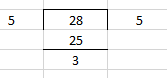∴ 28 / 5 =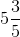(e) 19 / 6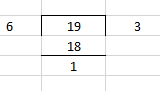∴ 19 / 6 =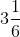(f) 35 / 9

∴ 35 / 9 =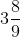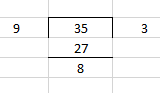3. Express the following as improper fractions:

(a)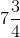(b)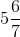(c)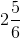(d)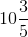(e)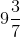(f)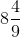Solutions:

(a) (7 × 4 + 3) / 4 = 31 / 4

∴ The improper form is 31 / 4

(b) (5 × 7 + 6) / 7 = 41 / 7

∴ The improper form is 41 / 7

(c) (2 × 6 + 5) / 6 = 17 / 6

∴ The improper form is 17 / 6

(d) (10 × 5 + 3) / 5 = 53 / 5

∴ The improper form is 53 / 5

(e) (9 × 7 + 3) / 7 = 66 / 7

∴ The improper form is 66 / 7

(f) (8 × 9 + 4) / 9 = 76 / 9

∴ The improper form is 76 / 9

## Exercise 7.3

1. Write the fractions. Are all these fractions equivalent?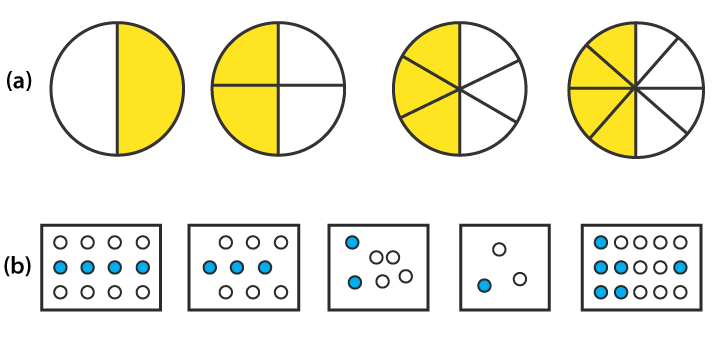Solutions:

(a)

(i) The shaded portion is 1 / 2

(ii) The shaded portion is 2 / 4 = (2 / 2) / (4 / 2) = 1 / 2

(iii) The shaded portion is 3 / 6 = (3 / 3) / (6 / 3) = 1 / 2

(iv) The shaded portion is 4 / 8 = (4 / 4) / (8 / 4) = 1 / 2

Hence, all fractions are equivalent.

(b)

(i) The shaded portion is 4 / 12 = (4 / 4) / (12 / 4) = 1 / 3

(ii)The shaded portion is 3 / 9 = (3 / 3) / (9 / 3) = 1 / 3

(iii) The shaded portion is 2 / 6 = (2 / 2) / (6 / 2) = 1 / 3

(iv) The shaded portion is 1 / 3

(v) The shaded portion is 6 / 15 = (6 / 3) / (15 / 3) = 2 / 5

All the fractions in their simplest form are not equal

Hence, they are not equivalent fractions.

2. Write the fractions and pair up the equivalent fractions from each row.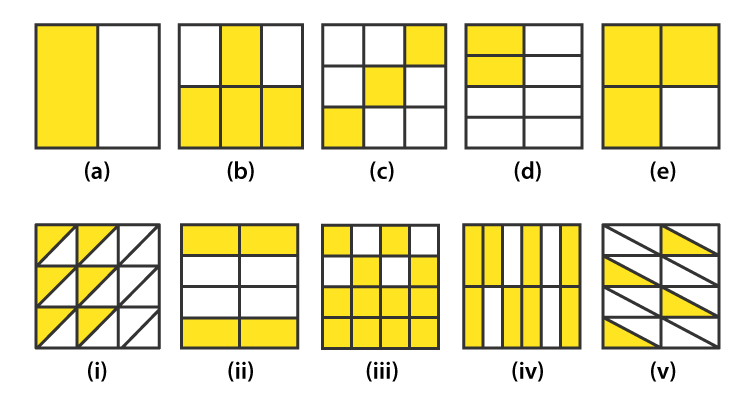Solutions:

(a) 1 / 2

(b) 4 / 6 = (4 / 2) / (6 / 2)

= 2 / 3

(c) 3 / 9 = (3 / 3) / (9 / 3)

= 1 / 3

(d) 2 / 8 = (2 / 2) / (8 / 2)

= 1 / 4

(e) 3 / 4

(i) 6 / 18 = (6 / 6) / (18 / 6)

= 1 / 3

(ii) 4 / 8 = (4 / 4) / (8 / 4)

= 1 / 2

(iii) 12 / 16 = (12 / 4) / (16 / 4)

= 3 / 4

(iv) 8 / 12 = (8 / 4) / (12 / 4)

= 2 / 3

(v) 4 / 16 = (4 / 4) / (16 / 4)

= 1 / 4

The following are the equivalent fractions

(a) and (ii) = 1 / 2

(b) and (iv) = 2 / 3

(c) and (i) = 1 / 3

(d) and (v) = 1 / 4

(e) and (iii) = 3 / 4

3. Replace  in each of the following by the correct number:

(a) 2 / 7 = 8 /

(b) 5 / 8 = 10 / ☐

(c) 3 / 5 = ☐ / 20

(d) 45 / 60 = 15 / ☐

(e) 18 / 24 = ☐ / 4

Solutions:

(a) Given

2 / 7 = 8 /

2 × ☐ = 7 × 8

☐ = (7 × 8) / 2

= 28

(b) Given

5 / 8 = 10 / ☐

☐ = (8 × 10) / 5

= 16

(c) Given

3 / 5 = ☐ / 20

☐ = (3 × 20) / 5

= 12

(d) Given

45 / 60 = 15 / ☐

☐ = (15 × 60) / 45

= 20

(e) Given

18 / 24 = ☐ / 4

☐ = (18 × 4) / 24

= 3

4. Find the equivalent fraction of 3 / 5 having

(a) denominator 20

(b) numerator 9

(c) denominator 30

(d) numerator 27

Solutions:

(a) We require denominator 20

Let M be the numerator of the fractions

∴ M / 20 = 3 / 5

5 × M = 20 × 3

M = (20 × 3) / 5

= 12

Therefore the required fraction is 12 / 20

(b) We require numerator 9

Let N be the denominator of the fractions

∴ 9 / N = 3 / 5

3 × N = 9 × 5

N = (9 × 5) / 3

= 15

Therefore the required fraction is 9 / 15

(c) We require denominator 30

Let D be the numerator of the fraction

∴ D / 30 = 3 / 5

5 × D = 3 × 30

D = (3 × 30) / 5

= 18

Therefore the required fraction is 18 / 30

(d) We require numerator 27

Let N be the denominator of the fraction

∴ 27 / N = 3 / 5

3 × N = 5 × 27

N = (5 × 27) / 3

= 45

Therefore the required fraction is 27 / 45

5. Find the equivalent fraction of 36 / 48 with

(a) numerator 9

(b) denominator 4

Solutions:

(a) Given numerator = 9

∴ 9 / D = 36 / 48

D × 36 = 9 × 48

D = (9 × 48) / 36

D = 12

Hence, the equivalent fraction is 9 / 12

(b) Given, denominator = 4

∴ N / 4 = 36 / 48

N × 48 = 4 × 36

N = (4 × 36) / 48

= 3

Hence, the equivalent fraction is 3 / 4

6. Check whether the given fractions are equivalent:

(a) 5 / 9, 30 / 54

(b) 3 / 10, 12 / 50

(c) 7 / 13, 5 / 11

Solutions:

(a) Given 5 / 9 and 30 / 54

We have 5× 54 = 270

9 × 30 = 270

5 × 54 = 9 × 30

Hence, 5 / 9 and 30 / 54 are equivalent fractions

(b) Given 3 / 10 and 12 / 50

We have 3 × 50 = 150

10 × 12 = 120

3 × 50 ≠ 10 × 12

Hence, 3 / 10 and 12 / 50 are not equivalent fractions

(c) Given 7 / 13 and 5 / 11

We have 7 × 11 = 77

5 × 13 = 65

7 × 11 ≠ 5 × 13

Hence, 7 / 13 and 5 / 11 are not equivalent fractions

7. Reduce the following fractions to the simplest form:

(a) 48 / 60

(b) 150 / 60

(c) 84 / 98

(d) 12 / 52

(e) 7 / 28

Solutions:

(a) 48 / 60 = (12 × 4) / (12 × 5)

= 4 / 5

(b) 150 / 60 = (30 × 5) / (30 × 2)

= 5 / 2

(c) 84 / 98 = (14 × 6) / (14 × 7)

= 6 / 7

(d) 12 / 52 = (3 × 4) / (13 × 4)

= 3 / 13

(e) 7 / 28 = 7 / (7 × 4)

= 1 / 4

8. Ramesh had 20 pencils, Sheelu had 50 pencils and Jamaal had 80 pencils. After 4 months, Ramesh used up 10 pencils, Sheelu used up 25 pencils and Jamaal used up 40 pencils. What fraction did each use up? Check if each has used up an equal fraction of her/his pencils?

Solutions:

Total number of pencils Ramesh had = 20

Number of pencils used by Ramesh = 10

∴ Fraction = 10 / 20 = 1 / 2

Total number of pencils Sheelu had = 50

Number of pencils used by Sheelu = 25

∴ Fraction = 25 / 50 = 1 / 2

Total number of pencils Jamaal had = 80

Number of pencils used by Jamaal = 40

∴ Fraction = 40 / 80 = 1 / 2

Yes, each has used up an equal fraction of pencils i.e 1 / 2

9. Match the equivalent fractions and write two more for each.

(i) 250 / 400 (a) 2 / 3

(ii) 180 / 200 (b) 2 / 5

(iii) 660 / 990 (c) 1 / 2

(iv) 180 / 360 (d) 5 / 8

(v) 220 / 550 (e) 9 / 10

Solutions:

(i) 250 / 400

= (5 × 50) / (8 × 50)

= 5 / 8

25 / 40 and 30 / 48 are two more fractions

(ii) 180 / 200

= (9 × 20) / (10 × 20)

= 9 / 10

18 / 20 and 27 / 30 are two more fractions

(iii) 660 / 990

= (2 × 330) / (3 × 330)

= 2 / 3

20 / 30 and 200 / 300 are two more fractions

(iv) 180 / 360

= (1 × 180) / (2 × 180)

= 1 / 2

20 / 40 and 30 / 60 are two more fractions

(v) 220 / 550

= (2 × 110) / (5 × 110)

= 2 / 5

20 / 50 and 40 / 100 are two more fractions

∴ The equivalent fractions are

(i) 250 / 100 = (d) 5 / 8

(ii) 180 / 200 = (e) 9 / 10

(iii) 660 / 990 = (a) 2 / 3

(iv) 180 / 360 = (c) 1 / 2

(v) 220 / 550 = (b) 2 / 5

## Exercise 7.4

1. Write shaded portion as a  fraction. Arrange them in ascending and descending order using the correct sign ‘’ between the fractions: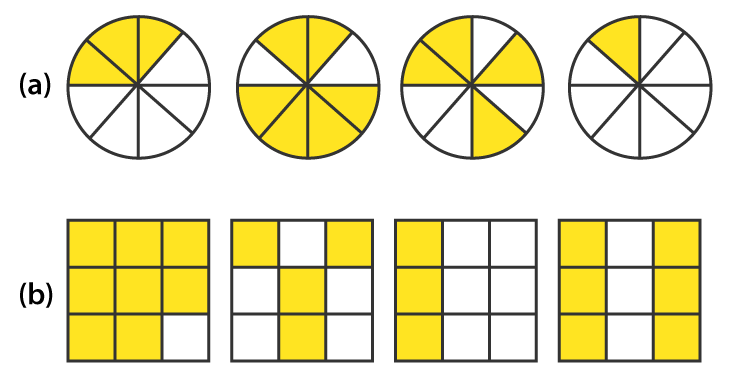(c) Show 2 / 6, 4 / 6, 8 / 6 and 6 / 6 on the number line. Put appropriate signs between the fractions given.

5 / 6 ☐ 2 / 6, 3 / 6 ☐ 0, 1 / 6 ☐ 6 / 6, 8 / 6 ☐ 5 / 6

Solutions:

(a) First circle shows 3 shaded parts out of 8 equal parts. Hence, the fraction is 3 / 8

Second circle shows 6 shaded parts out of 8 equal parts. Hence, the fraction is 6 / 8

Third circle shows 4 shaded parts out of 8 equal parts. Hence, the fraction is 4 / 8

Fourth circle shows 1 shaded part out of 8 equal parts. Hence, the fraction is 1 / 8

The arranged fractions are:

1 / 8 < 3 / 8 < 4 / 8 < 6 / 8

(b) First square shows 8 shaded parts out of 9 equal parts. Hence, the fraction is 8 / 9

Second square shows 4 shaded parts out of 9 equal parts. Hence, the fraction is 4 / 9

Third square shows 3 shaded parts out of 9 equal parts. Hence, the fraction is 3 / 9

Fourth square shows 6 shaded parts out of 9 equal parts. Hence, the fraction is 6 / 9

The arranged fractions are:

3 / 9 < 4 / 9 < 6 / 9 < 8 / 9

(c) Each unit length should be divided into 6 equal parts to represent the fractions 2 / 6, 4 / 6, 8 / 6 and

6 / 6 on number line. These fractions can be represented as follows: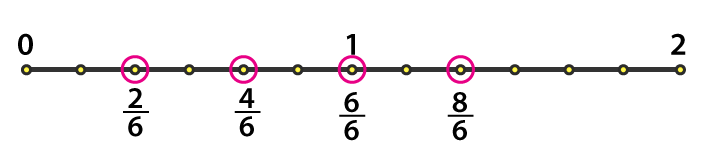5 / 6 > 2 / 6

3 / 6 > 0

1 / 6 < 6 / 6

8 / 6 > 5 / 6

2. Compare the fractions and put an appropriate sign.

(a) 3 / 6 ☐ 5 / 6

(b) 1 / 7 ☐ 1 / 4

(c) 4 / 5 ☐ 5 / 5

(d) 3 / 5 ☐ 3 / 7

Solutions:

(a) Here both fractions have same denominators. So, the fraction with greater numerator is the highest factor

∴ 3 / 6 < 5 / 6

(b) Multiply by 4

1 / 7 = (1 × 4) / (7 × 4)

= 4 / 28

Multiply by 7

1 / 4 = (1 × 7) / (4 × 7)

= 7 / 28

Here 4 < 7

∴ 1 / 7 < 1 / 4

(c) Here both fractions have same denominators. So, the fraction with the greater numerator is the highest factor

∴ 4 / 5 < 5 / 5

(d) Here both numerators are same. So, the fraction having less denominator will be the highest factor

∴ 3 / 7 < 3 / 5

3. Make five more such pairs and put appropriate signs.

Solutions:

(a) 5 / 8 < 6 / 8

Here, the denominators are same. So, the fraction having greater numerator is the highest factor

(ii) 5 / 8 > 2 / 8

Here, the denominators are same. So, the fraction having greater numerator is the highest factor

(iii) 6 / 13 > 6 / 18

Here, the numerators are same. So, the fraction having lesser denominator will be the highest factor

(iv) 5 / 25 > 3 / 25

Here, the denominators are same. So, the fraction having greater numerator is the highest factor

(v) 9 / 50 < 9 / 45

Here, the numerators are same. So, the fraction having lesser denominator will be the highest factor

4. Look at the figures and write ‘<’ or ‘>’, ‘=’ between the given pairs of fractions.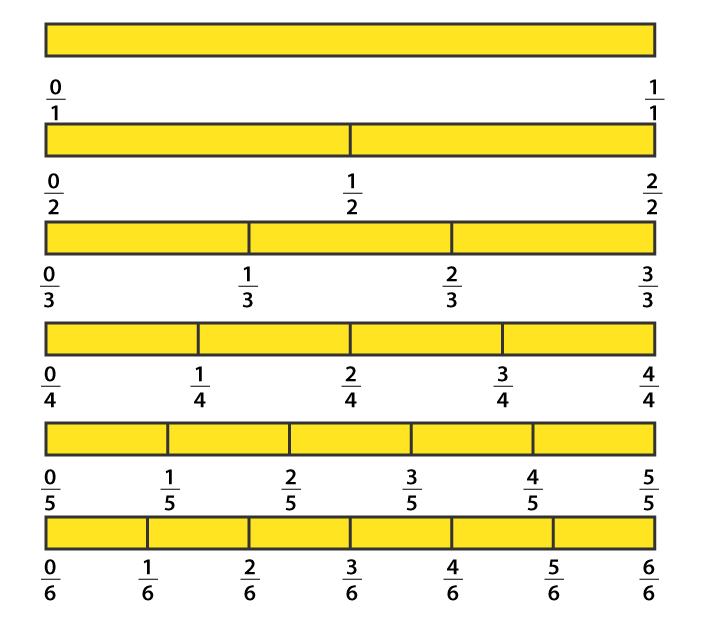(a) 1 / 6 ☐ 1 / 3

(b) 3 / 4 ☐ 2 / 6

(c) 2 / 3 ☐ 2 / 4

(d) 6 / 6 ☐ 3 / 3

(e) 5 / 6 ☐ 5 / 5

Solutions:

(a) Here, the numerators are same. So, the fraction having a lesser denominator is the greater

∴ 1 / 6 < 1 / 3

(b) 3 / 4 = (3 × 3) / (4 × 3)

= 9 / 12

2 / 6 = (2 × 2) / (6 × 2)

= 4 / 12

Between 4 / 12, 9 / 12

Both fractions have same denominators. So, the fraction having greater numerator will be the greater

∴ 9 / 12 > 4 / 12

3 / 4 > 2 / 6

(c) Here, the numerators are same. So, the fraction having a lesser denominator is the greater

∴ 2 / 3 > 2 / 4

(d) We get 6 / 6 = 1 and 3 / 3 = 1

So, 6 / 6 = 3 / 3

(e) Here, the numerators are same. So, the fraction having a lesser denominator is the greater

∴ 5 / 6 < 5 / 5

5. How quickly can you do this? Fill appropriate sign. ( ‘<’, ‘=’, ‘>’)

(a) 1 / 2 ☐ 1 / 5

(b) 2 / 4 ☐ 3 / 6

(c) 3 / 5 ☐ 2 / 3

(d) 3 / 4 ☐ 2 / 8

(e) 3 / 5 ☐ 6 / 5

(f) 7 / 9 ☐ 3 / 9

(g) 1 / 4 ☐ 2 / 8

(h) 6 / 10 ☐ 4 / 5

(i) 3 / 4 ☐ 7 / 8

(j) 6 / 10 ☐ 3 / 5

(k) 5 / 7 ☐ 15 / 21

Solutions:

(a) Here, the numerators are same. So, the fraction having a lesser denominator is the greater

∴ 1 / 2 > 1 / 5

(b) 2 / 4 = 1 / 2 and 3 / 6 = 1 / 2

∴ 2 / 4 = 3 / 6

(c) 3 / 5 = (3 × 3) / (5 × 3)

= 9 / 15

2 / 3 = (2 × 5) / 3 × 5)

= 10 / 15

Here, between 9 / 15 and 10 / 15 both have same denominators. Hence, the fraction having greater numerator will be the greater.

∴ 3 / 5 < 2 / 3

(d) Here, 2 / 8 = 1 / 4

As, 3 / 4 and 1 / 4 have same denominators. Hence, the fraction having greater numerator will be the greater

∴ 3 / 4 > 2 / 8

(e) Here, the denominators are same. So, the fraction having greater numerator will be the greater

∴ 3 / 5 < 6 / 5

(f) Here, the denominators are same. So, the fraction having a greater numerator will be the greater

∴ 7 / 9 > 3 / 9

(g) We know 2 / 8 = 1 / 4

Hence, 1 / 4 = 2 / 8

(h) 6 / 10 = (3 × 2) / (5 × 2)

= 3 / 5

Between 3 / 5 and 4 / 5

Both have same denominators. So, the fraction having greater numerator will be greater

∴ 6 / 10 < 4 / 5

(i) 3 / 4 = (3 × 2) / (4 × 2)

= 6 / 8

Between 6 / 8 and 7 / 8

Both have same denominators. So, the fraction having greater numerator will be greater

∴ 3 / 4 < 7 / 8

(j) 6 / 10 = (3 × 2) / (5 × 2)

= 3 / 5

∴ 6 / 10 = 3 / 5

(k) 5 / 7 = (5 × 3) / (7 × 3)

= 15 / 21

∴ 5 / 7 = 15 / 21

6. The following fractions represent just three different numbers. Separate them into three groups of equivalent fractions, by changing each one to its simplest form.

(a) 2 / 12 (b) 3 / 15 (c) 8 / 50 (d) 16 / 100 (e) 10 / 60 (f) 15 / 75

(g) 12 / 60 (h) 16 / 96 (i) 12 / 75 (j) 12 / 72 (k) 3 / 18 (l) 4 / 25

Solutions:

(a) 2 / 12 = (1 × 2) / (6 × 2)

= 1 / 6

(b) 3 / 15 = (1 × 3) / (5 × 3)

= 1 / 5

(c) 8 / 50 = (4 × 2) / (25 × 2)

= 4 / 25

(d) 16 / 100 = (4 × 4) / (25 × 4)

= 4 / 25

(e) 10 / 60 = (1 × 10) / (6 × 10)

= 1 / 6

(f) 15 / 75 = (1 × 15) / (5 × 15)

= 1 / 5

(g) 12 / 60 = (1 × 12) / (5 × 12)

= 1 / 5

(h) 16 / 96

= (1 × 16) / (6 × 16)

= 1 / 6

(i) 12 / 75 = (4 × 3) / (25 × 3)

= 4 / 25

(j) 12 / 72 = (1 × 12) / 6 × 12)

= 1 / 6

(k) 3 / 18 = (1 × 3) / (6 × 3)

= 1 / 6

(l) 4 / 25

Totally there are 3 groups of equivalent fractions.

1 / 6 = (a), (e), (h), (j), (k)

1 / 5 = (b), (f), (g)

4 / 25 = (c), (d), (i), (l)

7. Find answers to the following. Write and indicate how you solved them.

(a) Is 5 / 9 equal to 4 / 5

(b) Is 9 / 16 equal to 5 / 9

(c) Is 4 /5 equal to 16 / 20

(d) Is 1 / 15 equal to 4 / 30

Solutions:

(a) 5 / 9, 4 / 5

Convert these fractions into like fractions

5 / 9 = (5 / 9) × (5 / 5)

= 25 / 45

4 / 5 = (4 / 5) × (9 / 9)

= 36 / 45

∴ 25 / 45 ≠ 36 / 45

Hence, 5 / 9 is not equal to 4 / 5

(b) 9 / 16, 5 / 9

Convert into like fractions

9 / 16 = (9 / 16) × (9 / 9)

= 81 / 144

5 / 9 = (5 / 9) × (16 / 16)

= 80 / 144

∴ 81 / 144 ≠ 80 / 144

Hence, 9 / 16 is not equal to 5 / 9

(c) 4 / 5, 16 / 20

16 / 20 = (4 × 4) / (5 × 4)

= 4 / 5

∴ 4 / 5 = 16 / 20

Hence, 4 / 5 is equal to 16 / 20

(d) 1 / 15, 4 / 30

4 / 30 = (2 × 2) / (15 × 2)

= 2 / 15

∴ 1 / 15 ≠ 4 / 30

Hence, 1 / 15 is not equal to 4 / 30

8. Ila read 25 pages of a book containing 100 pages. Lalita read 2 / 5 of the same book. Who read less?

Solutions:

Total number of pages a book has = 100 pages

Lalita read = 2 / 5 × 100 = 40 pages

∴ Ila read less than Lalita.

9. Rafiq exercised for 3 / 6 of an hour, while Rohit exercised for 3 / 4 of an hour. Who exercised for a longer time?

Solutions:

Rafiq exercised = 3 / 6 of an hour

Rohit exercised = 3 / 4 of a hour

3 / 6, 3 / 4

Convert these into like fractions

3 / 6 = (3 × 2) / (6 × 2)

= 6 / 12

3 / 4 = (3 × 3) / (4 × 3)

= 9 / 12

Clearly, 9 / 12 > 6 / 12

∴ 3 / 4 > 3 / 6

Therefore Rohit exercised for a longer time than Rafiq.

10. In class A of 25 students, 20 passed with 60% or more marks; in another class B of 30 students, 24 passed with 60% or more marks. In which class was a greater fraction of students getting 60% or more marks?

Solutions:

Total number of students in Class A = 25

Students passed in first class in Class A = 20

Hence, fraction = 20 / 25

= 4 / 5

Total number of students in Class B = 30

Students passed in first class in Class B = 24

Hence, fraction = 24 / 30

= 4 / 5

∴ An equal fraction of students passed in first class in both the classes

## Exercise 7.5

1. Write these fractions appropriately as additions or subtractions: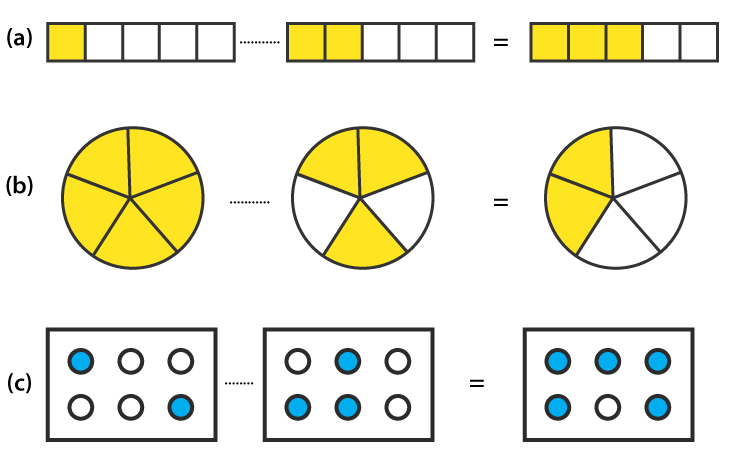Solutions:

(a) Total number of parts each rectangle has = 5

No. of shaded parts in first rectangle = 1 i.e 1 / 5

No. of shaded parts in second rectangle = 2 i.e 2 / 5

No. of shaded parts in third rectangle = 3 i.e 3 / 5

Clearly, fraction represented by third rectangle = Sum of the fractions represented by first and second rectangle

Hence, 1 / 5 + 2 / 5 = 3 / 5

(b) Total number of parts each circle has = 5

We may observe that the first, second, and third circles represent 5, 3, and 2 shaded parts out of 5 equal parts respectively. Clearly, the fraction represented by the third circle is the difference between the fractions represented by the first and second circles.

Hence, 5 / 5 – 3 / 5 = 2 / 5

(c) Here we may observe that the first, second and third rectangles represent 2, 3, and 5 shaded parts out of 6 equal parts respectively. Clearly, the fraction represented by the third rectangle is the sum of fractions represented by the first and second rectangles.

Hence, 2 / 6 + 3 / 6 = 5 / 6

2. Solve:

(a) 1 / 18 + 1 / 18

(b) 8 / 15 + 3 / 15

(c) 7 / 7 – 5 / 7

(d) 1 / 22 + 21 / 22

(e) 12 / 15 – 7 / 15

(f) 5 / 8 + 3 / 8

(g) 1 – 2 / 3 (1 = 3 / 3)

(h) 1 / 4 + 0 / 4

(i) 3 – 12 / 5

Solutions:

(a) 1 / 18 + 1 / 18

= (1 + 1) / 18

= 2 / 18

= 1 / 9

(b) 8 / 15 + 3 / 15

= (8 + 3) / 15

= 11 / 15

(c) 7 / 7 – 5 / 7

= (7 – 5) / 7

= 2 / 7

(d) 1 / 22 + 21 / 22

= (1 + 21) / 22

= 22 / 22

= 1

(e) 12 /15 – 7 / 15

= (12 – 7) / 15

= 5 / 15

= 1 / 3

(f) 5 / 8 + 3 / 8

= (5 + 3) / 8

= 8 / 8

= 1

(g) 1 – 2 / 3

= 3 / 3 – 2 / 3

= (3 – 2) / 3

= 1 / 3

(h) 1 / 4 + 0

= 1/ 4

(i) 3 – 12 / 5

= 15 / 5 – 12/ 5

= (15 – 12) / 5

= 3 / 5

3. Shubham painted 2 / 3 of the wall space in his room. His sister Madhavi helped and painted 1 / 3 of the wall space. How much did they paint together?

Solutions:

Wall space painted by Shubham in a room = 2 / 3

Wall space painted by Madhavi in a room = 1 / 3

Total space painted by both = (2 / 3 + 1 / 3)

= (2 + 1) / 3

= 3 / 3

= 1

∴ Shubham and Madhavi together painted 1 complete wall in a room.

4. Fill in the missing fractions.

(a) 7 / 10 – ▯ = 3 / 10

(b) ▯ – 3 / 21 = 5 / 21

(c) ▯ – 3 / 6 = 3 / 6

(d) ▯ + 5 / 27 = 12 / 27

Solutions:

(a) Given 7 / 10 – ▯ = 3 / 10

▯ = 7 / 10 – 3 / 10

▯ = (7 – 3) / 10

▯ = 4 / 10

▯ = 2 / 5

(b) Given ▯ – 3 / 21 = 5 / 21

▯ = 5 / 21 + 3 / 21

▯ = (5 + 3) / 21

▯ = 8 / 21

(c) Given ▯ – 3 / 6 = 3 / 6

▯ = 3 / 6 + 3 / 6

▯ = (3 + 3) / 6

▯ = 6 / 6

▯ = 1

(d) Given ▯ + 5 / 27 = 12 / 27

▯ = 12 / 27 – 5 /27

▯ = (12 – 5) / 27

▯ = 7 /27

5. Javed was given 5 / 7 of a basket of oranges. What fraction of oranges was left in the basket?

Solutions:

Fraction of oranges given to Javed = 5 / 7

Fraction of oranges left in the basket = 1 – 5 / 7

= 7 / 7 – 5 / 7

= (7 – 5) / 7

= 2 / 7

## Exercise 7.6

1. Solve

(a) 2 / 3 + 1 / 7

(b) 3 / 10 + 7 / 15

(c) 4 / 9 + 2 / 7

(d) 5 / 7 + 1 / 3

(e) 2 / 5 + 1 / 6

(f) 4 / 5 + 2 / 3

(g) 3 / 4 – 1 / 3

(h) 5 / 6 – 1 / 3

(i) 2 / 3 + 3 / 4 + 1 / 2

(j) 1/ 2 + 1 / 3 + 1 / 6

(k)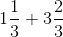(l)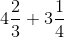(m) 16 / 5 – 7 / 5

(n) 4 / 3 – 1 / 2

Solutions:

(a) 2 / 3 + 1/ 7

Taking LCM

[(2 × 7) + (1 × 3)] / 21

= (14 + 3) / 21

= 17 / 21

(b) 3 / 10 + 7 / 15

Taking LCM 30

= [(3 × 3) + (7 × 2)] / 30

= (9 + 14) / 30

= 23 / 30

(c) 4 / 9 + 2/ 7

Taking LCM 63

= [(4 × 7) + (2 × 9)] / 63

= (28 + 18) / 63

= 46 / 63

(d) 5 / 7 + 1 / 3

Taking LCM 21

= [(5 × 3) + (1 × 7)] / 21

= (15 + 7) / 21

= 22 / 21

(e) 2 / 5 + 1 / 6

Taking LCM 30

= [(2 × 6) + (1 × 5)] / 30

= (12 + 5) / 30

= 17 / 30

(f) 4 / 5 + 2 / 3

Taking LCM 15

= [(4 × 3) + (2 × 5)] / 15

= (12 + 10) / 15

= 22 / 15

(g) 3 / 4 – 1 / 3

Taking LCM 12

= [(3 × 3) – (1 × 4)] / 12

= (9 – 4) / 12

= 5 / 12

(h) 5 / 6 – 1 / 3

Taking LCM 6

= [(5 × 1) – (1 × 2)] / 6

= (5 – 2) / 6

= 3 / 6

= 1 / 2

(i) 2 / 3 + 3 / 4 + 1 / 2

Taking LCM 12

= [(2 × 4) + (3 × 3) + (1 × 6)] / 12

= (8 + 9 + 6) / 12

= 23 / 12

(j) 1 / 2 + 1 / 3 + 1 / 6

Taking LCM 6

= [(1 × 3) + (1 × 2) + (1 × 1)] / 6

= (3 + 2 + 1) / 6

= 6 / 6

= 1

(k)= [(3 × 1) + 1] / 3 + [(3 × 3) + 2] / 3

= (3 + 1) / 3 + (9 + 2) / 3

= 4/ 3 + 11 / 3

= (4 + 11) / 3

= 15 / 3

= 5

(l)= [(3 × 4) + 2] / 3 + [(3 × 4) + 1] / 4

= 14 / 3 + 13 / 4

= [(14 × 4) + (13 × 3)] / 12

= (56 + 39) / 12

= 95 / 12

(m) 16 / 5 – 7 / 5

= (16 – 7) / 5

= 9 / 5

(n) 4 /3 – 1 / 2

Taking LCM 6

= [(4 × 2) – (1 × 3)] / 6

= (8 – 3) /6

= 5 / 6

2. Sarita bought 2 / 5 metres of ribbon and Lalita 3 /4 metres of ribbon. What is the total length of the ribbon they bought?

Solutions:

Ribbon length bought by Sarita = 2 / 5 metre

Ribbon length bought by Lalita = 3 / 4 metre

Total length of the ribbon bought by both of them = 2 / 5 + 3 / 4

Taking LCM 20

= [(2 × 4) + (3 × 5)] / 20

= (8 + 15) / 20

= 23 / 20 metre

∴ Total length of the ribbon bought by both Sarita and Lalita is 23 / 20 metre

3. Naina was given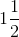piece of cake and Najma was given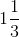piece of cake. Find the total amount of cake was given to both of them.

Solutions:

Fraction of cake Naina got == 3 / 2

Fraction of cake Najma got == 4 / 3

Total amount of cake given to both of them = 3 / 2 + 4 / 3

= [(3 × 3) + (4 × 2)] / 6

= (9 + 8) / 6

= 17 / 6

=4. Fill in the boxes:

(a) ▯ – 5 / 8 = 1 / 4

(b) ▯ – 1 / 5 = 1 / 2

(c) 1 / 2 – ▯ = 1 / 6

Solutions:

(a) ▯ – 5 / 8 = 1 / 4

▯ = 1 / 4 + 5 / 8

▯ = [(1 × 2 + 5)] / 8

▯ = 7 / 8

(b) ▯ – 1 / 5 = 1 / 2

▯ = 1 / 2 + 1 / 5

▯ = [(1 × 5) + (1 × 2)] / 10

▯ = (5 + 2) / 10

▯ = 7 / 10

(c) 1 / 2 – ▯ = 1 / 6

▯ = 1 / 2 – 1 / 6

▯ = [(1 × 3) – (1 × 1)] / 6

▯ = (3 – 1) / 6

▯ = 2 / 6

▯ 1 / 3

5. Complete the addition and subtraction box.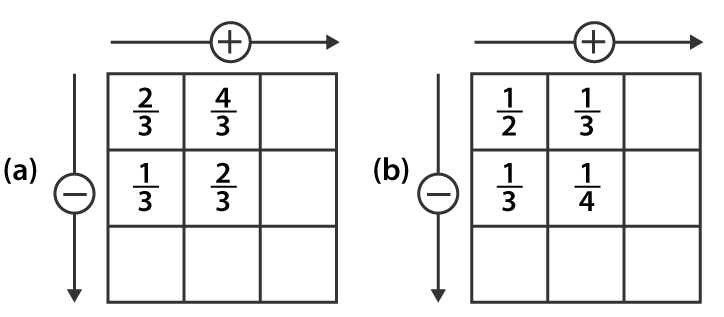Solutions:

(a) 2 / 3 + 4 / 3

= (2 + 4) / 3

= 6 / 3

= 2

1 / 3 + 2 / 3

= (1 + 2) / 3

= 3 / 3

= 1

2 / 3 – 1 / 3

= (2 – 1) / 3

= 1 / 3

4 / 3 – 2 / 3

= (4 – 2) / 3

= 2 / 3

1 / 3 + 2 / 3

= (1 + 2) / 3

= 3 / 3

= 1

Hence, the complete given box is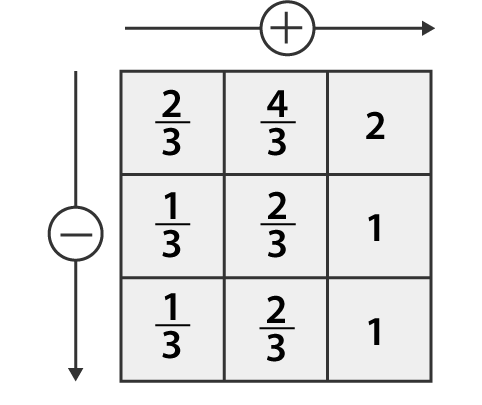(b) 1 / 2 + 1 / 3

= [(1 × 3) + (1 × 2)] / 6

= (3 + 2) / 6

= 5 / 6

1 / 3 + 1 / 4

= [(1 × 4) + (1 × 3)] / 12

= (4 + 3) / 12

= 7 / 12

1 / 2 – 1 / 3

= [(1 × 3) – (1 × 2)] / 6

= (3 – 2) / 6

= 1 / 6

1 / 3 – 1 / 4

= [(1 × 4) – (1 ×3)] / 12

= (4 – 3) / 12

= 1 / 12

1 / 6 + 1 / 12

= [(1 × 2) + 1] / 12

= (2 + 1) / 12

= 3 / 12

= 1 / 4

Hence, the complete given box is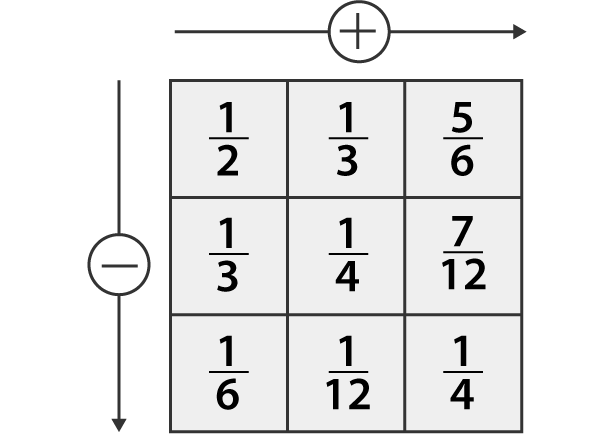6. A piece of wire 7 / 8 metre long broke into two pieces. One-piece was 1 / 4 metre long. How long is the other piece?

Solutions:

Total length of wire = 7 / 8 metre

Length of one piece of wire = 1 / 4 metre

Length of other piece of wire = Length of the original wire and this one piece of wire

= 7 / 8 – 1 / 4

= [(7 × 1) – (1 × 2)] / 8

= (7 – 2) / 8

= 5 / 8

∴ Length of the other piece of wire = 5 / 8 metre

7. Nandini’s house is 9 / 10 km from her school. She walked some distance and then took a bus for 1 / 2 km to reach the school. How far did she walk?

Solutions:

Distance of the school from house = 9 / 10 km

Distance she travelled by bus = 1 / 2 km

Distance walked by Nandini = Total distance of the school – Distance she travelled by bus

= 9 / 10 – 1 / 2

= [(9 × 1) – (1 × 5)] / 10

= (9 – 5) / 10

= 4 / 10

= 2 / 5 km

∴ Distance walked by Nandini is 2 / 5 km

8. Asha and Samuel have bookshelves of the same size partly filled with books. Asha’s shelf is 5 / 6 th full and Samuel’s shelf is 2/ 5 th full. Whose bookshelf is more full? By what fraction?

Solutions:

Fraction of Asha’s bookshelf = 5 / 6

Fraction of Samuel’s bookshelf = 2 / 5

Convert these fractions into like fractions

5 / 6 = 5 / 6 × 5 / 5

= (5 × 5) / (6 × 5)

= 25 / 30

2 / 5 = 2 / 5 × 6 / 6

= (2 × 6) / (5 × 6)

= 12 / 30

25 / 30 > 12 / 30

5 / 6 > 2 / 5

∴ Asha’s bookshelf is more full than Samuel’s bookshelf

Difference = 5 / 6 – 2 / 5

= 25 / 30 – 12 / 30

= 13 / 30

9. Jaidev takesminutes to walk across the school ground. Rahul takes 7 / 4 minutes to do the same. Who takes less time and by what fraction?

Solutions:

Time is taken by Jaidev to walk across the school ground == 11 / 5 minutes

Time taken by Rahul to walk across the school ground = 7 / 4 minutes

Convert these fractions into like fractions

11 / 5 = 11 / 5 × 4 / 4

= (11 × 4) / (5 × 4)

= 44 / 20

7 / 4 = 7 / 4 × 5 / 5

= (7 × 5) / (4 × 5)

= 35 / 20

Clearly, 44 / 20 > 35 / 20

11 / 5 > 7 / 4

∴ Rahul takes less time than Jaidev to walk across the school ground

Difference = 11 / 5 – 7 / 4

= 44 / 20 – 35 / 20

= 9 / 20

Hence, Rahul walks across the school ground by 9 / 20 minutes

I hope you like the information and notes given by us. This will help you a lot in your upcoming exams. Here we are giving ncert solutions to all of you students, through which all of you can score very well in your exams. In this post, we are giving you ncert solutions for class 6 maths. hope you benefit from it. You can download the pdf file of this ncert solutions.

 Whatsapp Group Join
 Telegram channel Join